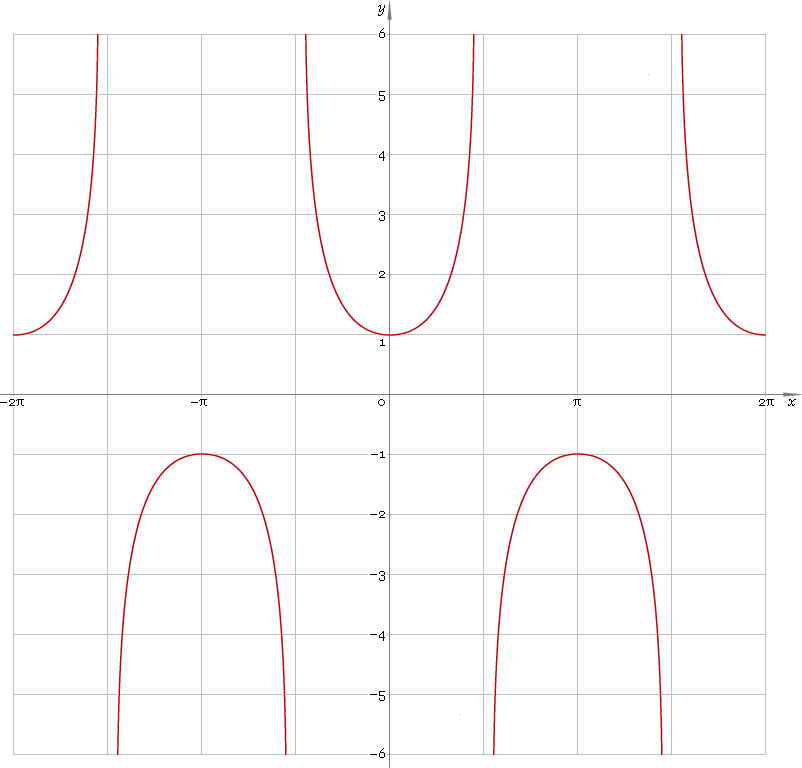The Art of Interface

# sec — trigonometric secant function

Category. Mathematics.

Abstract. Trigonometric secant: definition, graph, properties, identities and table of values for some angles.

## 1. Definition

Secant of the angle is ratio of the hypotenuse to adjacent leg.

## 2. Graph

Secant is 2π periodic function defined everywhere on real axis, except its singular points π/2 + πn, n=0, ±1, ±2, ... — so, function domain is (−π/2 + πn, π/2 + πn), n∈N. Its stalactite-stalagmite graph is depicted below — fig. 1.Fig. 1. Graph of the secant function y = secx.

Function codomain is entire real axis with gap in the middle: (−∞, −1]∪[1, +∞).

## 3. Identities

Base:

csc−2φ + sec−2φ = 1

and its consequences:

secφ = ±1 /√(1 − sin2φ)
secφ = ±√(1 + tan2φ)
secφ = ±√(1 + cot2φ) / cotφ
secφ = ±cscφ /√(csc2φ − 1)

By definition:

secφ ≡ 1 /cosφ

Properties — symmetry, periodicity, etc.:

sec−φ = cscφ
secφ = sec(φ + 2πn), where n = 0, ±1, ±2, ...
secφ = −sec(π − φ)
secφ = −sec(π + φ)
secφ = csc(π/2 + φ)

Sum of angles:

sec(φ + ψ + χ) = secφ secψ secχ / (1 − tanφ tanψ − tanφ tanχ − tanψ tanχ)

Some angles:

Angle φValue secφ
01
π/12√6 − √2
π/10√(50 − 10√5) /5
π/8√(2 − √2)
π/62√3 /3
π/5√5 − 1
π/4√2
3π/10√(50 + 10√5) /5
π/32
3π/8√(4 + 2√2)
2π/5√5 + 1
5π/12√6 + √2
Table 1. Secant for some angles.

## 4. Support

Trigonometric secant function sec is supported in:

Trigonometric secant function of the complex argument sec is supported in:

## 5. How to use

To calculate secant of the number:

``sec(-1);``

To calculate secant of the current result:

``sec(Rslt);``

To calculate secant of the angle φ in memory:

``sec(Mem[φ]);``